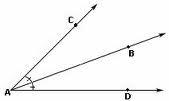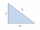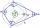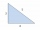# Angles of the triangle

ABC is a triangle. The size of the angles alpha, beta are in a ratio 4: 7. The angle gamma is greater than the angle alpha by a quarter of a straight angle. Determine angles of the triangle ABC.

α =  36 °
β =  63 °
γ =  81 °

### Step-by-step explanation:

7a = 4b; c = a+1/4 • 180; a+b+c=180

7•a = 4•b
c = a+1/4 • 180
a+b+c=180

7a-4b = 0
4a-4c = -180
a+b+c = 180

a = 36
b = 63
c = 81

Our linear equations calculator calculates it.Did you find an error or inaccuracy? Feel free to write us. Thank you!Tips to related online calculators
Do you have a linear equation or system of equations and looking for its solution? Or do you have a quadratic equation?

#### You need to know the following knowledge to solve this word math problem:

We encourage you to watch this tutorial video on this math problem:

## Related math problems and questions:

• Angles of a triangleIn triangle ABC, the angle beta is 15° greater than the angle alpha. The remaining angle is 30° greater than the sum of the angles alpha and beta. Calculate the angles of a triangle.
• Alfa beta gamaThe triangle's an interior angle beta is 10 degrees greater than the angle alpha and gamma angle is three times larger than the beta. Determine the size of the interior angles.
• In triangleIn triangle ABC, the magnitude of the internal angle gamma is equal to one-third of the angle alpha. The size of the angle beta is 80 degrees larger than the size of the gamma angle. Calculate the magnitudes of the interior angles of the triangle ABC.
• Alfa, beta, gamaIn the triangle ABC is the size of the internal angle BETA 8 degrees larger than the size of the internal angle ALFA and size of the internal angle GAMA is twice the size of the angle BETA. Determine the size of the interior angles of the triangle ABC.
• Angles in ratioThe size of the angles of the triangle are in ratio x: y = 7: 5 and the angle z is 42° lower than the angle y. Find size of the angles x, y, z.
• Angles ratioIn a triangle ABC true relationship c is less than b and b is less than a. Internal angles of the triangle are in the ratio 5:4:9. The size of the internal angle beta is:
• Angles in triangleThe triangle is ratio of the angles β:γ = 6:8. Angle α is 40° greater than β. What are the size of angles of the triangle?
• Triangle anglesThe angles α, β, γ in triangle ABC are in the ratio 6:2:6. Calculate size of angles.
• Circumferential angleVertices of the triangle ΔABC lay on the circle and divided into arcs in the ratio 7:8:7. Determine the size of the angles of the triangle ΔABC.
• Internal anglesFind the internal angles of the triangle ABC if the angle at the vertex C is twice the angle at the B and the angle at the vertex B is 4 degrees smaller than the angle at the vertex A.
• Angle at the apexIn an isosceles triangle, the angle at the apex is 30° greater than the angle at the base. How big are the internal angles?What size have angles in quadrilateral (4-gon) if they are in a ratio of 8: 9: 10: 13?Calculate the remaining internal and external angles of a triangle, if you know the internal angle γ (gamma) = 34 degrees and one external angle is 78 degrees and 40 '. Determine what kind of triangle it is from the size of its angles.In a thirteen sided polygon, the sum of five angles is 1274°, four of the eight angles remaining are equal and the other four are 18° less than each of the equal angles. Find the angles. .The second angle of a triangle is the same size as the first angle. The third angle is 12 degrees larger than the first angle. How large are the angles?The angles of the triangle ABC make an arithmetic sequence with the largest angle γ=83°. What sizes have other angles in a triangle?In a triangle, ABC, the interior angle at vertex C is twice the internal angle at point A. The outer angle at point B measured 117 degrees. How big is the external angle at vertex A?